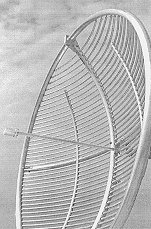Search IntMath
Close

By M. BourneA parabolic antenna uses the quadratic function

Quadratic equations are used in many areas of science and engineering. The path of a projectile (e.g. a cannon ball) is (almost) parabolic, and we use a quadratic equation to find out where the projectile is going to hit.

Parabolic antennas are another application.

Real mathematics is about modelling situations that occur naturally, and using the model to understand what is happening, or maybe to predict what will happen in future.

The quadratic equation is often used in modelling because it is a beautifully simple curve.

### Applications and Related Sections in Interactive Mathematics

The parabola is closely related to the quadratic equation

Complex Numbers arise out of the problem where we cannot find the square root of a negative number.

We can see quadratic equations used in integration by partial fractions

Curve sketching (an application of differentiation)

Areas under parabolic curves and Simpson's Rule (applications of integration)

Solving second-order homogeneous linear differential equations

Finding the intersection point of two circles

## What's in this Chapter?

1. Solving Quadratic Equations by Factoring, where we learn how to use factorising to find the value of x in problems like: x^2− 7x + 10 = 0

2. Completing the Square, which introduces the concept behind the quadratic formula.

4. The Graph of the Quadratic Function is a parabola, and this helps us to visualize the solution for a quadratic.

5. Equations in Quadratic Form shows how some complicated equations can be reduced to a quadratic formula and then easily solved.

We begin the chapter with a reminder of factoring and some examples of Solving Quadratic Equations by Factoring »

## Problem SolverThis tool combines the power of mathematical computation engine that excels at solving mathematical formulas with the power of GPT large language models to parse and generate natural language. This creates math problem solver thats more accurate than ChatGPT, more flexible than a calculator, and faster answers than a human tutor. Learn More.